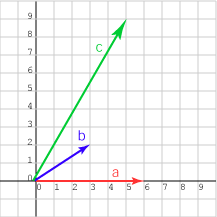c is much longer vector than b; it is likely that a · c is the larger.

# Length Affects Dot ProductThe answer is not only likely, but correct.

• a is (6,  0)T
• b is (3,  2)T
• c is (5,  9)T

The dot products are:

• a · b   = 18.0
• a · c   = 30.00

There are two properties tangled up in the dot product :

• the angle between the input vectors
• the length of the input vectors

We would like to get just the angle by somehow removing the influence of length.

### QUESTION 3:

What is the normal method for removing the influence of length?